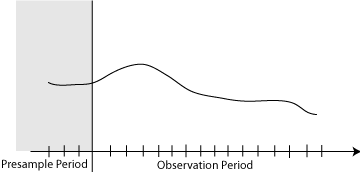## Presample Values for regARIMA Model Estimation

Presample data comes from time points before the beginning of the observation period. In Econometrics Toolbox™, you can specify your own presample data or use generated presample data.In regression models with ARIMA errors, the distribution of the current innovation (εt) is conditional on historic information (Ht). Historic information can include past unconditional disturbances or past innovations, i.e., Ht = {ut – 1,εt – 1,ut – 2,εt – 2,...,u0,ε0,u–1,ε–1,...}. However, the software does not include past responses (yt) nor past predictors (Xt) in Ht. For example, in a regression model with ARIMA(2,1,1) errors, you can write the error model in several ways:

• $\left(1-{\varphi }_{1}L-{\varphi }_{2}{L}^{2}\right)\left(1-L\right){u}_{t}=\left(1+{\theta }_{1}L\right){\epsilon }_{t}.$

• $\left(1-L-{\varphi }_{1}\left(L-{L}^{2}\right)-{\varphi }_{2}\left({L}^{2}-{L}^{3}\right)\right){u}_{t}=\left(1+{\theta }_{1}L\right){\epsilon }_{t}.$

• ${u}_{t}={u}_{t-1}+{\varphi }_{1}\left({u}_{t-1}-{u}_{t-2}\right)+{\varphi }_{2}\left({u}_{t-2}-{u}_{t-3}\right)+{\epsilon }_{t}+{\theta }_{1}{\epsilon }_{t-1}.$

• ${\epsilon }_{t}={u}_{t}-{u}_{t-1}-{\varphi }_{1}\left({u}_{t-1}-{u}_{t-2}\right)-{\varphi }_{2}\left({u}_{t-2}-{u}_{t-3}\right)-{\theta }_{1}{\epsilon }_{t-1}.$

The last equation implies that:

• The first innovation in the series (ε1) depends on the history H1 = {u–2,u–1,u0,ε0}. H1 is not observable nor inferable from the regression model.

• The second innovation in the series (ε2) depends on the history H2 = {u–1,u0,u1,ε1}. The software can infer u1 and ε1, but not the others.

• The third innovation in the series (ε3) depends on the history H3 = {u0,u1,u2,ε2}. The software can infer u1, u2, and ε1, but not u0.

• The rest of the innovations depend on inferable unconditional disturbances and innovations.

Therefore, the software requires three presample unconditional disturbances to initialize the autoregressive portion, and one presample innovation to initialize the moving average portion.

The degrees of the compound autoregressive and moving average polynomials determine the number of past unconditional disturbances and innovations that εt depends on. The compound autoregressive polynomial includes the seasonal and nonseasonal autoregressive, and seasonal and nonseasonal integration polynomials. The compound moving average polynomial includes the seasonal and nonseasonal moving average polynomials. In the example, the degree of the compound autoregressive polynomial is `P` = 3, and the degree of the moving average polynomial is `Q` = 1. Therefore, the software requires three presample unconditional disturbances and one presample innovation.

If you do not have presample values (or do not supply them), then, by default, the software backcasts for the necessary presample unconditional disturbances, and sets the necessary presample innovations to 0.

Another option to obtain presample unconditional disturbances is to partition the data set into a presample portion and estimation portion:

1. Partition the data such that the presample portion contains at least `max(P,Q)` observations. The software uses the most recent `max(P,Q)` observations and ignores the rest.

2. For the presample portion, regress yt onto Xt.

3. Infer the residuals from the regression model. These are the presample unconditional disturbances.

4. Pass the presample unconditional disturbances (`U0`) and the estimation portion of the data into `estimate`.

This option results in a loss of sample size. Note that when comparing multiple models using likelihood-based measures of fit (such as likelihood ratio tests or information criteria), then the data must have the same estimation portions, and the presample portions must be of equal size.

If you plan on specifying presample values, then you must specify at least the number necessary to initialize the series.

You can specify both presample unconditional disturbances and innovations, one or the other, or neither.